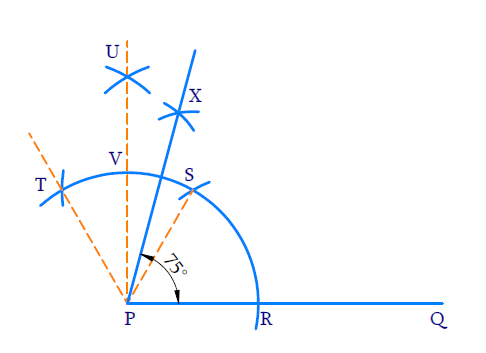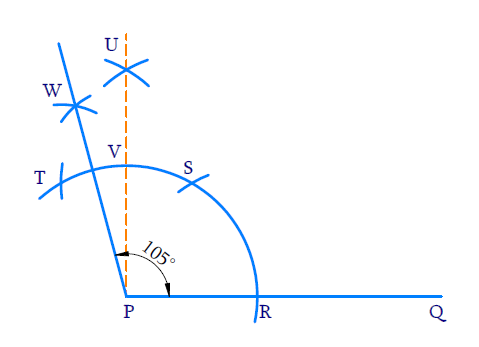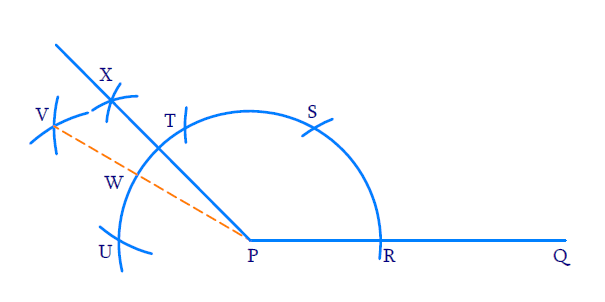In the verge of coronavirus pandemic, we are providing FREE access to our entire Online Curriculum to ensure Learning Doesn't STOP!

# Ex.11.1 Q4 Constructions Solution - NCERT Maths Class 9

Go back to  'Ex.11.1'

## Question

Construct the following angles and verify by measuring them by a protractor:

(i) $$75^{\circ}$$

(ii) $$105^{\circ}$$

(iii) $$135^{\circ}$$

Video Solution
Constructions
Ex 11.1 | Question 4

## Text Solution

$${\rm{(i)}}\;\;75^{\circ}$$

Reasoning:

We need to construct two adjacent angles of $$60^{\circ}$$.The second angle should be bisected twice to get a $$15^{\circ}$$ angle.

\begin{align}75^{\circ}&=60^{\circ}+15^{\circ} \\ 15^{\circ}&=\frac{30^{\circ}}{2}=\frac{60^{\circ}}{2} \div \frac{1}{2}\end{align}

Steps of Construction:(i) Draw ray $$PQ$$.

(ii) To construct an angle of $$60^{\circ}$$ .

With $$P$$ as centre and any radius draw a wide arc to intersect $$PQ$$ at $$R$$ With $$R$$ as centre and same radius draw an arc to intersect the initial arc at $$S$$ .$$\angle \mathrm{SPR}=60^{\circ}$$

(iii) To construct adjacent angle of $$60^{\circ}$$ with $$S$$ as center and same radius draw an arc to $$T$$ intersect the initial arc.

(iv) To bisect $$\angle \mathrm{SPT}$$

With $$T$$ and $$S$$ as centres and same radius draw arcs to bisect each other at $$U$$ Join $$U$$ and $$P$$. \begin{align}\angle \mathrm{UPS}=\frac{1}{2} \angle \mathrm{TPS}=30^{\circ}\end{align}

(v) To bisect $$\angle \mathrm{UPS}$$

With $$V$$ and $$S$$ as centers and radius greater than half of $$VS$$ draw arcs to intersect each other at $$X$$

\begin{align} \angle {XPS} &=\frac{1}{2} \angle {UPS} \\ &=\frac{1}{2} \times 30^{0} \\ &=15^{\circ} \\ \angle {XPQ} &=\angle {XPS}+\angle {SPQ} \\ &=15^{\circ}+60^{\circ} \end{align}

$${\rm{(ii)}}\;\;105^{\circ}$$

Reasoning:

We need to construct two adjacent angles of $$60^{\circ}$$ . In the second angle we need to bisect it to get two $$30^{\circ}$$ angles. The second $$30^{\circ}$$ angle should be bisected again to get a $$15^{\circ}$$ angle. Together we can make an angle of $$105^{\circ}$$ .

\begin{align} 105^{\circ} &=60^{\circ}+45^{\circ} \\ 45^{\circ} &=60^{\circ}+30^{\circ}+15^{\circ} \end{align}

Steps of Construction:(i) Draw ray $$PQ$$

(ii) To construct an angle of $$60^{\circ}$$.

With $$P$$ as centre and any radius draw a wide arc to intersect $$PQ$$ at $$R$$. With $$R$$ as centre and same radius draw an arc to intersect the initial arc at $$S$$. $$\angle \mathrm{SPR}=60^{\circ}$$

(iii) To construct an adjacent angle of $$60^{\circ}$$ with $$S$$ as the center and same radius as before draw an arc to intersect the initial arc at $$T$$. $$\angle \mathrm{TPS}=60^{\circ}$$

(iv) To bisect $$\angle \mathrm{TPS}$$

With $$T$$ and $$S$$ as centres and same radius draw arcs to bisect each other at $$U$$. Join $$U$$ and $$P$$

\begin{align}\angle \text{UPS = }\frac{1}{2}\angle \text{TPS }=\frac{1}{2}\times {{60}^{0}}\text{=30}{}^\circ \end{align}

(v)To bisect $$\angle \text{UPT}$$

With $$T$$ and $$V$$ as centers and radius greater than half of $$TV,$$draw arcs to intersect each other at $$W$$. Join $$P$$ and $$W$$.

\begin{align} \angle {WPU} &=\frac{1}{2} \angle {UTP} \\ &=\frac{1}{2} \times 30^{\circ} \\ &=15^{\circ} \\ \angle {WPR} &=\angle {WPU}+\angle {UPS}+\angle {SPR} \\ &=15^{\circ}+30^{\circ}+60^{\circ} \\ &=105^{\circ} \end{align}

$${\rm{(iii)}}\;\;135^{\circ}$$

Reasoning:

We need to construct three adjacent angles of $$60^{\circ}$$ each. The third angle should be bisected twice successively to get an angle of $$15^{\circ}$$ . Together we will get an angle of $$135^{\circ}$$ .

\begin{align} 135^{\circ} &=15^{\circ}+60^{\circ}+60^{\circ} \\ 15^{\circ} &=\frac{60^{\circ}}{2} \div \frac{1}{2} \end{align}

Steps of Construction:(i) Draw ray$$PQ$$

(ii) To construct an angle of $$60^{\circ}$$ .

With $$P$$ as the center and any radius draw an arc to intersect $$PQ$$ at $$R$$.$$\angle \mathrm{SPR}=60^{\circ}$$

(iii) To construct adjacent angle of $$60^{\circ}$$

With $$S$$ as the center and same radius as before draw an arc to intersect the initial arc at $$T$$. $$\angle \mathrm{TPS}=60^{\circ}$$

(iv) To construct the second adjacent angle of $$60^{\circ}$$

With $$T$$ as centre and some radius as before draw an arc to intersect the initial arc at $$U$$

$\angle \mathrm{UPT}=60^{\circ}$

(v) To bisect $$\angle \mathrm{UPT}$$

With $$T$$ and $$U$$ as centers and same radius as before draw an arc to intersect each other at $$V$$ $$\angle \mathrm{VPT}=\angle \mathrm{VPU}=30^{\circ}$$

(vi) To bisect $$\angle \mathrm{VPT}$$

With $$W$$ and $$T$$ as centers and radius greater than half of $$WT$$ draw arcs to intersect each other at $$X$$.

\begin{align}XPT&=\angle XPV \\&=7\frac{1}{2}\angle V\text{PT}\\&=\frac{1}{2}\times {{30}^\circ}=\,15{}^\circ \\ \\ \angle XPQ &= \angle \text{XPT + }\angle TPS + \angle SPR \\&= 15{}^\circ + 60{}^\circ + 60{}^\circ \\&=135{}^\circ \,\,\, \\\end{align}

Learn from the best math teachers and top your exams

• Live one on one classroom and doubt clearing
• Practice worksheets in and after class for conceptual clarity
• Personalized curriculum to keep up with school